Open in App
Not now

# Customized Debugging in Sublime Text using C++ for Competitive Programming

• Difficulty Level : Medium
• Last Updated : 13 Jan, 2022
• Competitive Programming is a mental sport that enables us to code a given problem under provided constraints. The purpose of this article is to guide every individual on how they can debug their code efficiently during a contest.

Time is something that is precious and it matters a lot during a coding contest. When writing a code, errors do come and programmers often tend to spend a lot of time debugging it.  Often programmers deal with complex data structures during a contest and it is required to debug them in the given time constraint.

This article focuses on how to debug C++ source code efficiently in Sublime Text (IDE) during a contest and save time. First of all, it is required to set up the file structure of our Sublime Text. Below are the steps to set up the file structure of Sublime Text.

Step 1: Open the Sublime Text and follow the below steps:

1. Create three files:

• file.cpp: The file to write the code.
• inputf.txt: The file where we will be giving the input.
• outputf.txt: The file where the output will be displayed.

2. Now, perform the following steps:

• Select View > Layout > Columns: 3. This will create three columns in the workspace. Move the three files into three columns.
• Select View > Groups > Max Columns : 2 : input.txt and output.txt will get stacked in a single column.

Your Sublime Text would look similar to this: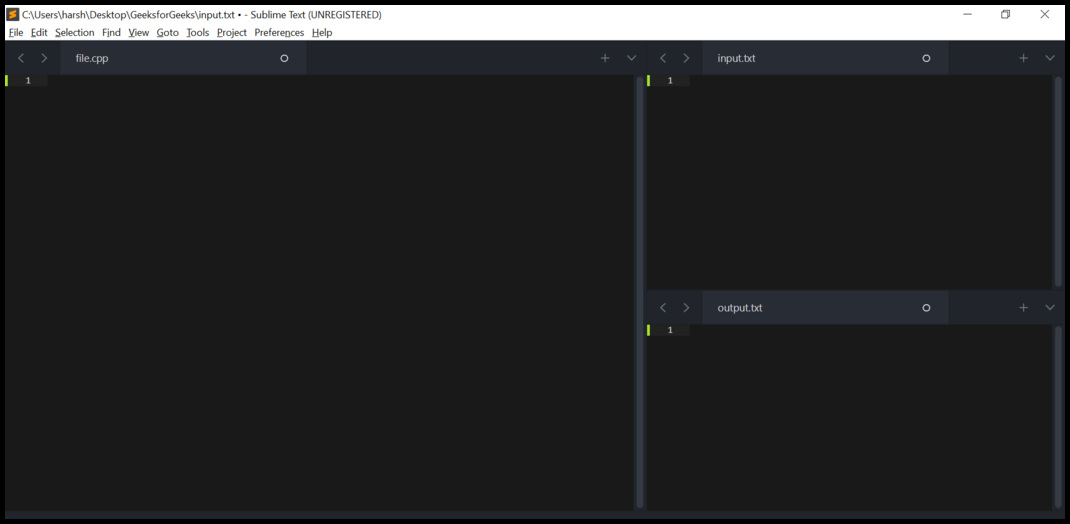Sublime Text

Step 2: Create a local function outside the main function. It is used to take input from the input.txt file and display output in the output.txt file. Below is the C++ code snippet for the same.

## C++

 `// Declare this function outside``// the main function``void` `local()``{``  ``// In case of online judges (like``  ``// codechef, codeforces etc) these``  ``// lines will be skipped. In other``  ``// words these lines would be executed``  ``// in Sublime Text only``  ``#ifndef ONLINE_JUDGE` `  ``freopen``(``"input.txt"``, ``"r"``, stdin);``  ``freopen``(``"output.txt"``, ``"w"``, stdout);` `  ``// ONLINE_JUDGE``  ``#endif``}`

Step 3: Call from the main function:

```// Call from the main function
local();```

By combining the above steps our complete program would be:

## C++

 `// C++ program to implement``// the above approach``#include ``using` `namespace` `std;` `// Local function``void` `local()``{``  ``#ifndef ONLINE_JUDGE``  ``freopen``(``"input.txt"``,``          ``"r"``, stdin);``  ``freopen``(``"output.txt"``,``          ``"w"``, stdout);``  ` `  ``// ONLINE_JUDGE``  ``#endif``}` `// Driver code``int` `main()``{``  ``local();``  ``return` `0;``}`

Step 4: Now the IDE would look similar to this: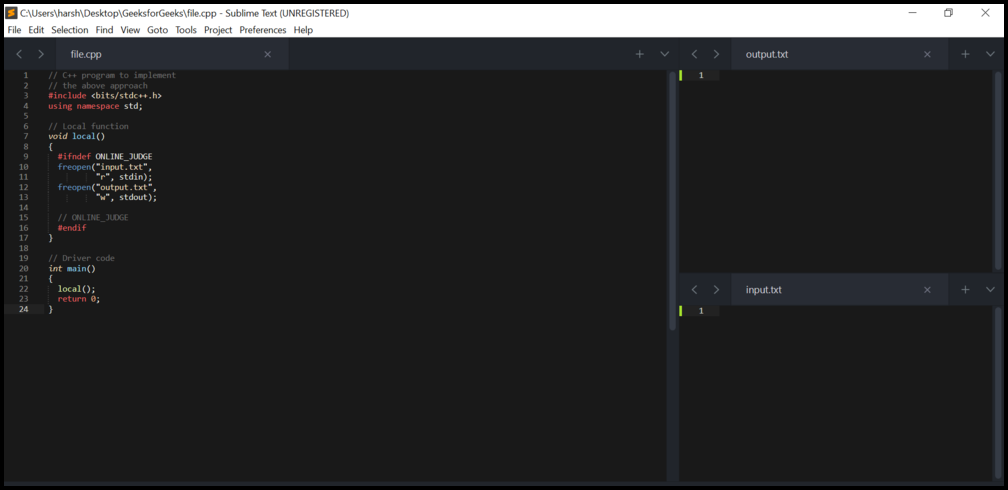Sublime Text

Debugging using print function:

Create a print function in our program whenever we need to print a variable or any data structure like vector, set, map, etc. Below is the C++ program to implement the same approach:

## C++

 `// C++ program to implement``// the above approach``#include ``using` `namespace` `std;` `// Driver code``int` `main()``{``  ``// Initializing a vector``  ``vector<``int``> vect = {2, 4, 10,``                      ``12, 17};` `  ``// First operation``  ``for` `(``auto``& x : vect)``  ``{``    ``if` `(x % 2 == 0)``      ``x += 10;``    ``else``      ``x -= 10;``  ``}` `  ``// Second operation``  ``for` `(``auto``& x : vect)``    ``x += 2;` `  ``// Third operation``  ``for` `(``auto``& x : vect)``    ``x += 20;``}`

Suppose something went wrong in our logic due to which desired output is not obtained during a contest and hence to check the status of vector after the first operation, one can create a print function outside the main function which accepts a vector.

## C++

 `// print function outside the``// main function``void` `print(vector<``int``>& vect)``{``  ``cout << ``"vect "` `<< ``' '``;``  ``cout << ``'['` `<< ``' '``;` `  ``// Print vector elements``  ``for` `(``auto` `x : vect)``  ``{``    ``cout << x << ``' '``;``  ``}``  ``cout << ``']'``;``}`

Whenever there is a need to check the vector elements one can call the print() function by passing the vector as an argument to the print function.

```// Calling print function from main

print(vect);```

Below is the complete C++ program to illustrate how to implement the above concept:

## C++

 `// C++ program to implement``// the above concept``#include ``using` `namespace` `std;` `// Print function for debugging``void` `print(vector<``int``>& vect)``{``  ``cout << ``"vect "` `<< ``' '``;``  ``cout << ``'['` `<< ``' '``;` `  ``// Print vector elements``  ``for` `(``auto` `x : vect)``  ``{``    ``cout << x << ``' '``;``  ``}``  ``cout << ``']'``;``}` `// Driver code``int` `main()``{``  ``// Initializing a vector``  ``vector<``int``> vect = {2, 4, 10,``                      ``12, 17};` `  ``// First operation``  ``for` `(``auto``& x : vect)``  ``{``    ``if` `(x % 2 == 0)``      ``x += 10;``    ``else``      ``x -= 10;``  ``}` `  ``// Printing vect elements after``  ``// applying first operation``  ``// Checking the status of vect as``  ``// a part of debugging``  ``print(vect);` `  ``// Second operation``  ``for` `(``auto``& x : vect)``    ``x += 2;` `  ``// Third operation``  ``for` `(``auto``& x : vect)``    ``x += 20;` `  ``int` `finalAnswer = 0;``  ``for` `(``auto` `x : vect)``    ``finalAnswer += x;` `  ``// Print the final answer``  ``cout << ``"\nFinal Answer: "` `<<``            ``finalAnswer;``  ``return` `0;``}`

Output

```vect  [ 12 14 20 22 7 ]

• For the same data structure but having different data types there is a need to create multiple print functions. For example, there is a vector of integer type and a vector of string type, then in order to print elements, it is required to create two print functions outside the main function. One print function will accept a vector of integer type and another print function will accept a vector of string type.
• Contents of the vector would be printed along with the desired values in the same output.txt file which might be confusing for us.
• There is a need to comment statements that are used to call a print function from the main function, before eventually submitting the source code file to the online judge (Codeforces, Spoj, Codechef, etc).

Debugging using template:

In the above method, the data type of vector is hardcoded. The template can be used in C++. A template is a simple and yet very powerful tool in C++. The simple idea is to pass data type as a parameter so that there is no need to write the same code (print function) for different data types. Below is the C++ code snippet for the template:

## C++

 `// One print function works for``// all data types.  This would work``// even for user defined types if``// operator '>' is overloaded``template` `<``typename` `T>` `void` `print(vector vect)``{``    ``// body``}`

Below is the complete C++ program to illustrate the above concept:

## C++

 `// C++ program to implement``// the above approach``#include ``using` `namespace` `std;` `// Using template so that this``// function works for all data``// types``template` `<``typename` `T> ``void` `print(``          ``vector& vect)``{``  ``cout << ``"vect "` `<< ``' '``;``  ``cout << ``'['` `<< ``' '``;` `  ``for` `(``auto` `x : vect)``  ``{``    ``cout << x << ``' '``;``  ``}``  ``cout << ``']'``;``  ``cout << ``'\n'``;``}` `// Driver code``int` `main()``{``  ``vector<``int``> vect1 = {2, 4, 10,``                       ``12, 17};` `  ``for` `(``auto``& x : vect1)``  ``{``    ``if` `(x % 2 == 0)``      ``x += 10;``    ``else``      ``x -= 10;``  ``}` `  ``// Printing vect1 elements``  ``print(vect1);` `  ``// Initializing a vector of``  ``// string type``  ``vector vect2 = {``"Geeks"``,``                          ``"for"``, ``"Geeks"``};` `  ``// Printing vect2 elements``  ``print(vect2);` `  ``// Modifying vect2``  ``// push back string "is great"``  ``vect2.push_back(``"is the great"``);` `  ``// Printing vect2 after modification``  ``print(vect2);` `  ``int` `finalAnswer = 0;``  ``for` `(``auto` `x : vect1)``    ``finalAnswer += x;``  ``cout << ``"Final Answer: "` `<<``           ``finalAnswer;``  ``return` `0;``}`

Output

```vect  [ 12 14 20 22 7 ]
vect  [ Geeks for Geeks ]
vect  [ Geeks for Geeks is the great ]

A similar thing can be done with any data structure like a set, multiset, pairs, etc. Below is the implementation using the set:

## C++

 `// C++ program to implement``// the above approach``#include ``using` `namespace` `std;` `// Using template so that this``// function works for all data``// types``template` `<``typename` `T> ``void` `print(``          ``set& set1)``{``  ``cout << ``"set "` `<< ``' '``;``  ``cout << ``'['` `<< ``' '``;` `  ``for` `(``auto` `x : set1)``  ``{``    ``cout << x << ``' '``;``  ``}``  ``cout << ``']'``;``  ``cout << ``'\n'``;``}` `// Driver code``int` `main()``{``  ``// Declaring a set``  ``set<``int``> set1;` `  ``// Inserting elements in the set``  ``for` `(``int` `i = 0; i < 10; i++)``    ``set1.insert(i);` `  ``// Printing set1 elements``  ``print(set1);` `  ``// Declaring another set of``  ``// string type``  ``set set2;` `  ``// Inserting elements in the set``  ``set2.insert(``"GeeksforGeeks"``);` `  ``// Printing set2 elements``  ``print(set2);` `  ``int` `finalAnswer = 0;``  ` `  ``for` `(``auto` `x : set1)``    ``finalAnswer += x;``  ``cout << ``"Final Answer: "` `<<``           ``finalAnswer;``  ``return` `0;``}`

Output

```set  [ 0 1 2 3 4 5 6 7 8 9 ]
set  [ GeeksforGeeks ]

• The above method is not very efficient as each time before submitting the program there is a need to comment print statements inside the main function.
• Elements of the data structure would be printed along with other desired values in the same output.txt file which might be confusing for us.

Debugging using cerr:

The idea is to use the combination of cerr  (error stream) and file handling in the program. Create a separate file (error.txt) and use cerr stream instead of cout stream. Finally, with the help of file handling, print the status of data structure in the error.txt file.

Step 1: Firstly add the following snippets outside the main function:

• To print a variable we can create a print function with a template definition just above the function:

## C++

 `//Template definition``template` `<``typename` `T>` `//Function to print the variable``void` `print(T x)``{``  ``// Using error stream to print``  ``// the variable``  ``cerr << x;``}`

• To print vector elements we can create a print function with a template definition just above the function:

## C++

 `// Template definition``template` `<``typename` `T>` `// Function to print the elements``// of the vector``void` `print(vector& a)``{``  ``cerr << ``'['` `<< ``' '``;` `  ``for` `(``auto` `x : a)``  ``{``    ``// Same to print a variable (Function``    ``// Overloading)``    ``print(x);` `    ``cerr << ``' '``;``  ``}``  ``cerr << ``']'``;``}`

• To print set elements arranged in non-descending order we can create a print function with a template definition just above the function:

## C++

 `//Template definition``template` `<``typename` `T>` `// Function to print elements of the``// set arranged in non-descending order``void` `print(set& a)``{``  ``cerr << ``'['` `<< ``' '``;` `  ``for` `(``auto` `x : a)``  ``{``    ``// Same as printing a variable``    ``// (Function Overloading)``    ``print(x);` `    ``cerr << ``' '``;``  ``}``  ``cerr << ``']'``;``}`

• To print set elements arranged in non-ascending order we can create a print function with a template definition just above the function:

## C++

 `template` `<``typename` `T>` `// Function to print the set elements``// arranged in non-ascending order``void` `print(set >& a)``{``  ``cerr << ``'['` `<< ``' '``;` `  ``for` `(``auto` `x : a)``  ``{``    ``// same as printing a variable``    ``// (Function Overloading)``    ``print(x);` `    ``cerr << ``' '``;``  ``}``  ``cerr << ``']'``;``}`

• To print unordered set elements, we can create a print function with a template definition just above the function:

## C++

 `// Template definition``template` `<``typename` `T>` `// Function to print unordered``// set elements``void` `print(unordered_set& a)``{``  ``cerr << ``'['` `<< ``' '``;` `  ``for` `(``auto` `x : a)``  ``{``    ``// Same as printing a variable``    ``// Using the concept of function``    ``// overloading``    ``print(x);``    ` `    ``cerr << ``' '``;``  ``}``  ``cerr << ``']'``;``}`

• To print map elements, we can create a print function with a template definition just above the function:

## C++

 `//Template definition``template` `<``typename` `T, ``typename` `V>` `//Function to print map elements``// arranged in non-descending order``void` `print(map& a)``{``  ``cerr << ``"[ "``;` `  ``for` `(``auto` `i : a)``  ``{``    ``// Same as variable using the``    ``// concept of function overloading``    ``print(i);` `    ``cerr << ``" "``;``  ``}``  ``cerr << ``"]"``;``}`

• To print unordered map elements, we can create a print function with a template definition just above the function:

## C++

 `//Template definition``template` `<``typename` `T, ``typename` `V>` `//Function to print unordered map elements``void` `print(unordered_map& a)``{``  ``cerr << ``"[ "``;``  ``for` `(``auto` `i : a)``  ``{``    ``// Same as variable using the``    ``// concept of function overloading``    ``print(i);``    ` `    ``cerr << ``" "``;``  ``}``  ``cerr << ``"]"``;``}`

• To print multiset elements arranged in non-descending order, we can create a print function with a template definition just above the function:

## C++

 `//Template definition``template` `<``typename` `T>` `//Function to print multiset elements``// arranged in non-descending order``void` `print(multiset& a)``{``  ``cerr << ``'['` `<< ``' '``;` `  ``for` `(``auto` `x : a)``  ``{``    ``// Same as variable using the``    ``// concept of function overloading``    ``print(x);` `    ``cerr << ``' '``;``  ``}``  ``cerr << ``']'``;``}`

• To print multiset elements, arranged in non-ascending order we can create a print function with a template definition just above the function:

## C++

 `//Template definition``template` `<``typename` `T>` `//Function to print elements of a``// multiset arranged in non-ascending order``void` `print(multiset >& a)``{``  ``cerr << ``'['` `<< ``' '``;` `  ``for` `(``auto` `x : a)``  ``{``    ``// Same as variable using the``    ``// concept of function overloading``    ``print(x);` `    ``cerr << ``' '``;``  ``}``  ``cerr << ``']'``;``}`

• To print unordered set elements, we can create a print function with a template definition just above the function:

## C++

 `// Template definition``template` `<``typename` `T>` `// Print function to print unordered``// set elements``void` `print(unordered_set& a)``{``  ``cerr << ``'['` `<< ``' '``;` `  ``for` `(``auto` `x : a)``  ``{``    ``// Same as variable using the``    ``// concept of function overloading``    ``print(x);` `    ``cerr << ``' '``;``  ``}``  ``cerr << ``']'``;``}`

• To print vector of vectors elements, we can create a print function with a template definition just above the function:

## C++

 `// Template definition``template` `<``typename` `T>` `// Function to print vector of``// vectors elements``void` `print(vector >& a)``{``  ``cerr << ``"[ "``;` `  ``for` `(``auto` `i : a)``  ``{``    ``// Same as variable using the``    ``// concept of function overloading``    ``print(i);``    ` `    ``cerr << ``" "``;``  ``}``  ``cerr << ``"]"``;``}`

• To print a pair of elements, we can create a print function with a template definition just above the function:

## C++

 `// Template definition``template` `<``typename` `T, ``typename` `V>` `// Function to print elements of a pair``void` `print(pair x)``{``  ``// Sam as printing the variable using``  ``// the concept of function overloading``  ``print(x.ff);``  ` `  ``cerr << ``':'``;` `  ``// Same as variable using the concept``  ``// of function overloading``  ``print(x.ss);``}`

• To print a pair of vectors elements, we can create a print function with a template definition just above the function:

## C++

 `// Template definition``template` `<``typename` `T, ``typename` `V>` `// Function to print vector of``// pairs elements``void` `print(vector >& a)``{``  ``cerr << ``'['` `<< ``' '``;` `  ``for` `(``auto` `x : a)``  ``{``    ``// Same as printing a variable using``    ``// the concept of function overloading``    ``print(x.ff);``    ``cerr << ``":"``;` `    ``// Same as printing a variable using``    ``// the concept of function overloading``    ``print(x.ss);``    ``cerr << ``' '``;``  ``}``  ``cerr << ``']'``;``}`

Step 2: Create one more new file (error.txt) and make sure it is in the same folder.

error.txt file: All the elements of the data structures that we have mentioned in the above snippets would be printed in this text file only without affecting the output.txt file.

Note that we want to write in this file using error stream (cerr). Again help from ifndef and endif preprocessor directives can be taken.

## C++

 `// We want to skip writing on error.txt``// file when online judge (Codechef,``// Codeforces, etc) is defined``#ifndef ONLINE_JUDGE``    ``freopen``(``"error.txt"``, ``"w"``, stderr);` `// ONLINE_JUDGE``#endif`

After adding the above lines in our local function, the complete function becomes:

## C++

 `// Now local function would look like:``void` `local()``{``  ``#ifndef ONLINE_JUDGE``  ``freopen``(``"input.txt"``, ``"r"``, stdin);``  ``freopen``(``"output.txt"``, ``"w"``, stdout);``  ` `  ``// ONLINE_JUDGE``  ``#endif` `  ``// It basically means that these``  ``// statements (Between ifndef and``  ``// endif) would be skipped / ignored``  ``// if ONLINE_JUDGE is defined We don't``  ``// need to comment "local();" statement``  ``// in our function while submitting our``  ``// source code file to online judges.``  ``// It would be handled automatically` `  ``#ifndef ONLINE_JUDGE``  ``freopen``(``"error.txt"``, ``"w"``, stderr);``  ` `  ``// ONLINE_JUDGE``  ``#endif``}`

Step 3: Also, we don’t want to comment debug(data_structure) statements while submitting the source code file to online judges. In simple words, there is a need to figure out a way so that debug functions will work for the sublime text (IDE) but they would be skipped/ignored for online judges.
Again, this can be achieved by using ifndef and endif preprocessor directives again in the source code.

## C++

 `// If online judge is defined``#ifndef ONLINE_JUDGE``#define debug(x)                                          ``cerr << #x << ``"  "``;                                   ``print(x);                                            ``cerr << ``'\n'``;` `// If Online Judge is not defined``#else``#define debug(x)``#endif`

Now the IDE would look similar to this: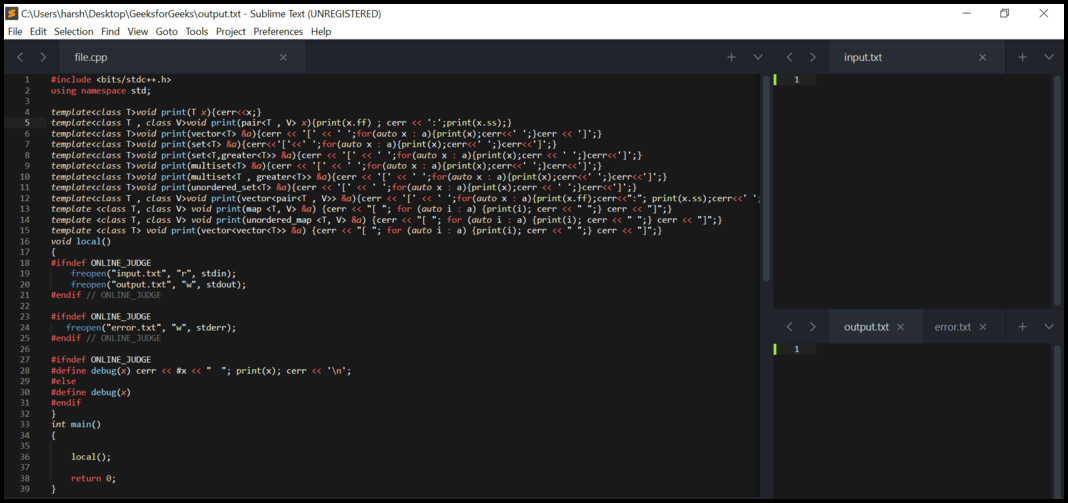Step 4: Whenever there is a need to check the status of any data structure, the following call can be made:

```// Calling from the main function
debug(dataStructure);```

Step 5: Below is the implementation of the above method:

Example 1:

## C++

 `/* It is recommended below snippets in``   ``your template file of competitive programming */``#include ``using` `namespace` `std;` `// Debugging Functions` `template``<``class` `T>``void` `print(T x)``{``  ``cerr << x;``}``template``<``class` `T, ``class` `V>``         ``void` `print(pair x)``{``  ``print(x.ff);``  ``cerr << ``':'``;``  ``print(x.ss);``}``template``<``class` `T>``         ``void` `print(vector &a)``{``  ``cerr << ``'['` `<< ``' '``;``  ``for``(``auto` `x : a)``  ``{``    ``print(x);``    ``cerr << ``' '``;``  ``}``  ``cerr << ``']'``;``}``template``<``class` `T>``         ``void` `print(set &a)``{``  ``cerr << ``'['` `<< ``' '``;``  ``for``(``auto` `x : a)``  ``{``    ``print(x);``    ``cerr << ``' '``;``  ``}``  ``cerr << ``']'``;``}``template``<``class` `T>``         ``void` `print(set> &a)``{``  ``cerr << ``'['` `<< ``' '``;``  ``for``(``auto` `x : a)``  ``{``    ``print(x);``    ``cerr << ``' '``;``  ``}``  ``cerr << ``']'``;``}``template``<``class` `T>``         ``void` `print(multiset &a)``{``  ``cerr << ``'['` `<< ``' '``;``  ``for``(``auto` `x : a)``  ``{``    ``print(x);``    ``cerr << ``' '``;``  ``}``  ``cerr << ``']'``;``}``template``<``class` `T>``         ``void` `print(multiset> &a)``{``  ``cerr << ``'['` `<< ``' '``;``  ``for``(``auto` `x : a)``  ``{``    ``print(x);``    ``cerr << ``' '``;``  ``}``  ``cerr << ``']'``;``}``template``<``class` `T>``         ``void` `print(unordered_set &a)``{``  ``cerr << ``'['` `<< ``' '``;``  ``for``(``auto` `x : a)``  ``{``    ``print(x);``    ``cerr << ``' '``;``  ``}``  ``cerr << ``']'``;``}``template``<``class` `T, ``class` `V>``         ``void` `print(vector> &a)``{``  ``cerr << ``'['` `<< ``' '``;``  ``for``(``auto` `x : a)``  ``{``    ``print(x.ff);``    ``cerr << ``":"``;``    ``print(x.ss);``    ``cerr << ``' '``;``  ``}``  ``cerr << ``']'``;``}``template` `<``class` `T, ``class` `V>``          ``void` `print(map &a)``{``  ``cerr << ``"[ "``;``  ``for` `(``auto` `i : a)``  ``{``    ``print(i);``    ``cerr << ``" "``;``  ``}``  ``cerr << ``"]"``;``}``template` `<``class` `T, ``class` `V>``          ``void` `print(unordered_map &a)``{``  ``cerr << ``"[ "``;``  ``for` `(``auto` `i : a)``  ``{``    ``print(i);``    ``cerr << ``" "``;``  ``}``  ``cerr << ``"]"``;``}``template` `<``class` `T>``          ``void` `print(vector> &a)``{``  ``cerr << ``"[ "``;``  ``for` `(``auto` `i : a)``  ``{``    ``print(i);``    ``cerr << ``" "``;``  ``}``  ``cerr << ``"]"``;``}` `void` `local()``{``  ``// ONLINE_JUDGE``  ``#ifndef ONLINE_JUDGE``  ``freopen``(``"input.txt"``, ``"r"``, stdin);``  ``freopen``(``"output.txt"``, ``"w"``, stdout);``  ``#endif` `  ``// ONLINE_JUDGE``  ``#ifndef ONLINE_JUDGE``  ``freopen``(``"error.txt"``, ``"w"``, stderr);``  ``#endif` `  ``#ifndef ONLINE_JUDGE``  ``#define debug(x)``  ``cerr << #x << ``"  "``;``  ``print(x);``  ``cerr << ``'\n'``;``  ``#else``  ``#define debug(x)``  ``#endif``}` `// Driver code``int` `main()``{``  ``local();` `  ``// Number of elements in the vector``  ``int` `n;` `  ``// Taking input from the user``  ``// through input.txt file``  ``cin >> n;` `  ``// Declaring a vector of integer``  ``// type of size n``  ``vector<``int``> vect1(n);` `  ``// Initializing the vector   ``  ``for``(``int` `i = 0 ; i < n ; i++)``    ``cin >> vect1[i];` `  ``// Modifying the vector``  ``for` `(``auto``& x : vect1)``  ``{``    ``if` `(x % 2 == 0)``      ``x += 10;``    ``else``      ``x -= 10;``  ``}` `  ``// Printing vect1 elements``  ``// It will be printed in error.txt``  ``// file using cerr stream``  ``debug(vect1);` `  ``// Initializing a vector of string type``  ``vector vect2 = {``"Geeks"``, ``"for"``, ``"Geeks"``};` `  ``// Printing vect2 elements``  ``// It will be printed in error.txt``  ``// file using cerr stream``  ``debug(vect2);` `  ``// Modifying vect2``  ``// push back string "is great"``  ``vect2.push_back(``"is the great"``);` `  ``// Printing vect2 after modification``  ``// It will be printed in error.txt``  ``// file using cerr stream``  ``debug(vect2);` `  ``// Calculating the answer``  ``int` `finalAnswer = 0;``  ``for` `(``auto` `x : vect1)``    ``finalAnswer += x;` `  ``// Finally, printing answer in output.txt``  ``// file using cout stream``  ``cout << ``"Final Answer: "` `<< finalAnswer;` `  ``return` `0;``}`

file.cpp file: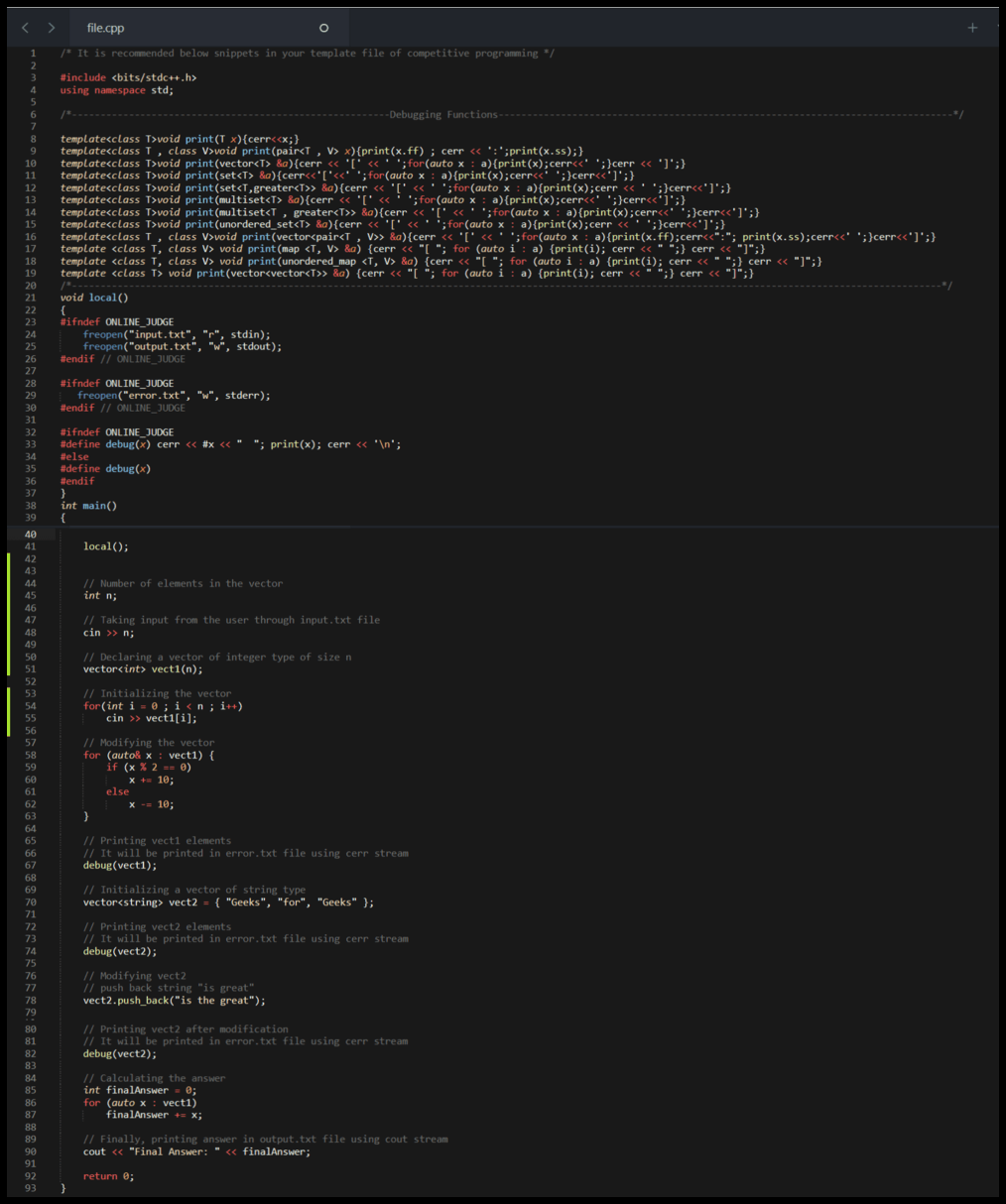input.txt file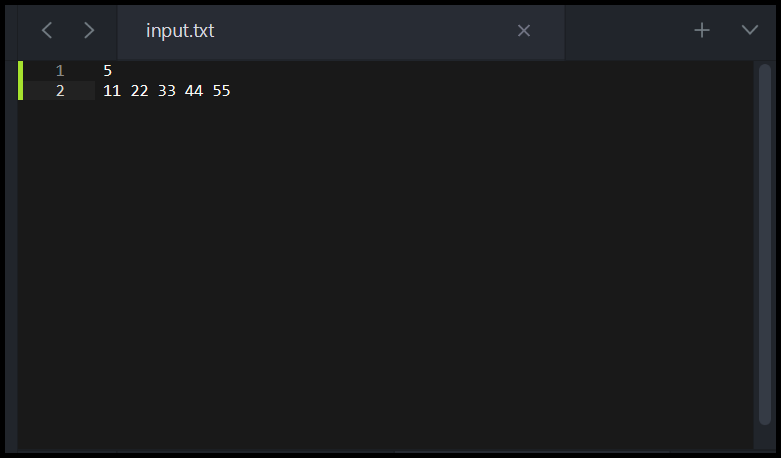output.txt file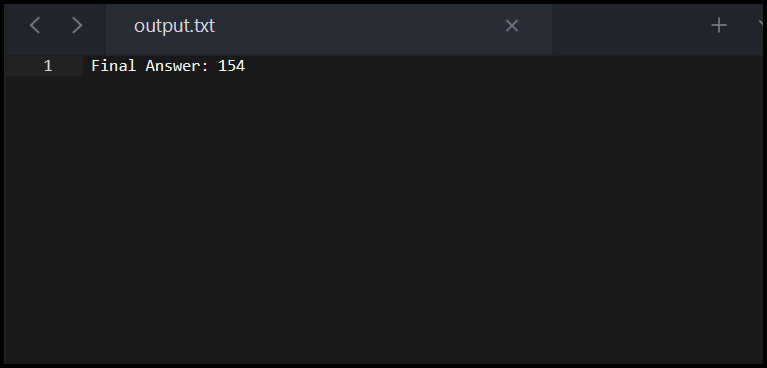error.txt file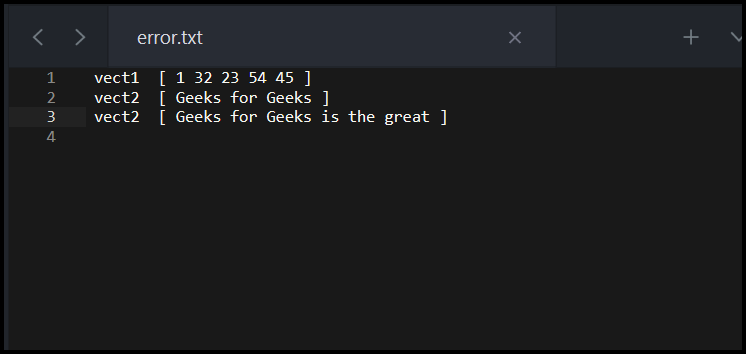Example 2:

## C++

 `/* It is recommended below snippets in``   ``your template file of competitive programming */` `#include ``using` `namespace` `std;` `// Debugging Functions``template``<``class` `T>``void` `print(T x)``{``  ``cerr << x;``}``template``<``class` `T , ``class` `V>``         ``void` `print(pair x)``{``  ``print(x.ff);``  ``cerr << ``':'``;``  ``print(x.ss);``}``template``<``class` `T>``         ``void` `print(vector &a)``{``  ``cerr << ``'['` `<< ``' '``;``  ``for``(``auto` `x : a)``  ``{``    ``print(x);``    ``cerr << ``' '``;``  ``}``  ``cerr << ``']'``;``}``template``<``class` `T>``         ``void` `print(set &a)``{``  ``cerr << ``'['` `<< ``' '``;``  ``for``(``auto` `x : a)``  ``{``    ``print(x);``    ``cerr << ``' '``;``  ``}``  ``cerr << ``']'``;``}``template``<``class` `T>``         ``void` `print(set> &a)``{``  ``cerr << ``'['` `<< ``' '``;``  ``for``(``auto` `x : a)``  ``{``    ``print(x);``    ``cerr << ``' '``;``  ``}``  ``cerr << ``']'``;``}``template``<``class` `T>``         ``void` `print(multiset &a)``{``  ``cerr << ``'['` `<< ``' '``;``  ``for``(``auto` `x : a)``  ``{``    ``print(x);``    ``cerr << ``' '``;``  ``}``  ``cerr << ``']'``;``}``template``<``class` `T>``         ``void` `print(multiset> &a)``{``  ``cerr << ``'['` `<< ``' '``;``  ``for``(``auto` `x : a)``  ``{``    ``print(x);``    ``cerr << ``' '``;``  ``}``  ``cerr << ``']'``;``}``template``<``class` `T>``         ``void` `print(unordered_set &a)``{``  ``cerr << ``'['` `<< ``' '``;``  ``for``(``auto` `x : a)``  ``{``    ``print(x);``    ``cerr << ``' '``;``  ``}``  ``cerr << ``']'``;``}``template``<``class` `T, ``class` `V>``         ``void` `print(vector> &a)``{``  ``cerr << ``'['` `<< ``' '``;``  ``for``(``auto` `x : a)``  ``{``    ``print(x.ff);``    ``cerr << ``":"``;``    ``print(x.ss);``    ``cerr << ``' '``;``  ``}``  ``cerr << ``']'``;``}``template` `<``class` `T, ``class` `V>``          ``void` `print(map &a)``{``  ``cerr << ``"[ "``;``  ``for` `(``auto` `i : a)``  ``{``    ``print(i);``    ``cerr << ``" "``;``  ``}``  ``cerr << ``"]"``;``}``template` `<``class` `T, ``class` `V>``          ``void` `print(unordered_map &a)``{``  ``cerr << ``"[ "``;``  ``for` `(``auto` `i : a)``  ``{``    ``print(i);``    ``cerr << ``" "``;``  ``}``  ``cerr << ``"]"``;``}``template` `<``class` `T>``          ``void` `print(vector> &a)``{``  ``cerr << ``"[ "``;``  ``for` `(``auto` `i : a)``  ``{``    ``print(i);``    ``cerr << ``" "``;``  ``}``  ``cerr << ``"]"``;``}` `void` `local()``{``  ``// ONLINE_JUDGE``  ``#ifndef ONLINE_JUDGE``  ``freopen``(``"input.txt"``, ``"r"``, stdin);``  ``freopen``(``"output.txt"``, ``"w"``, stdout);``  ``#endif` `  ``// ONLINE_JUDGE``  ``#ifndef ONLINE_JUDGE``  ``freopen``(``"error.txt"``, ``"w"``, stderr);``  ``#endif` `  ``#ifndef ONLINE_JUDGE``  ``#define debug(x)``  ``cerr << #x << ``"  "``;``  ``print(x);``  ``cerr << ``'\n'``;``  ``#else``  ``#define debug(x)``  ``#endif``}` `// Driver code``int` `main()``{``  ``local();` `  ``// Number of elements in the set``  ``int` `n;` `  ``// Taking input from the user``  ``// through input.txt file``  ``cin >> n;` `  ``// Declaring a set of integers``  ``set<``int``> set1;` `  ``for``(``int` `i = 0 ; i < n ; i++)``  ``{``    ``int` `number;` `    ``// Taking input from the user``    ``// through input.txt file``    ``cin >> number;``    ` `    ``//Inserting in the set``    ``set1.insert(number);  ``  ``}   `  `  ``// Erasing from the set``  ``if``(!set1.empty())``  ``{``    ``// erasing the first element``    ``// of the set``    ``set1.erase(set1.begin());``  ``}` `  ``// Printing set1 elements``  ``// It will be printed in error.txt``  ``// file using cerr stream``  ``debug(set1);` `  ``// Declaring another set``  ``set set2;` `  ``// Inserting in the set``  ``set2.insert(``"GeeksforGeeks"``);` `  ``// Printing set2 elements``  ``// It will be printed in error.txt file``  ``// using cerr stream``  ``debug(set2);` `  ``// Inserting in set``  ``// Insert the string "is great"``  ``set2.insert(``"Geek"``);` `  ``// Printing set2 elements after``  ``// inserting into the set, It will``  ``// be printed in error.txt file``  ``// using cerr stream``  ``debug(set2);` `  ``// Calculating the answer``  ``int` `finalAnswer = 0;``  ``for` `(``auto` `x : set1)``    ``finalAnswer += x;` `  ``// Finally, printing answer in output.txt``  ``// file using cout stream``  ``cout << ``"Final Answer: "` `<< finalAnswer;` `  ``return` `0;``}`

file.cpp file: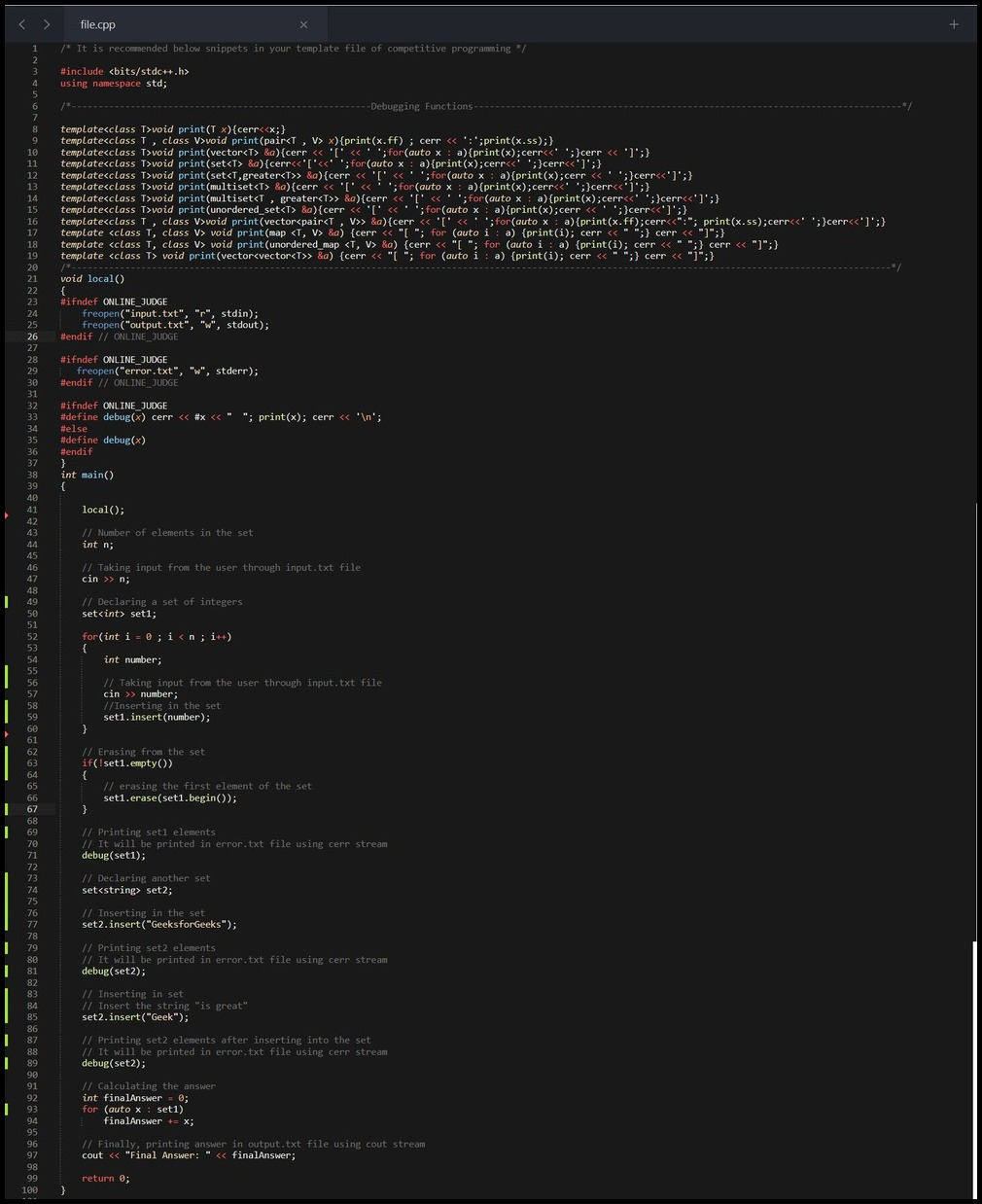input.txt file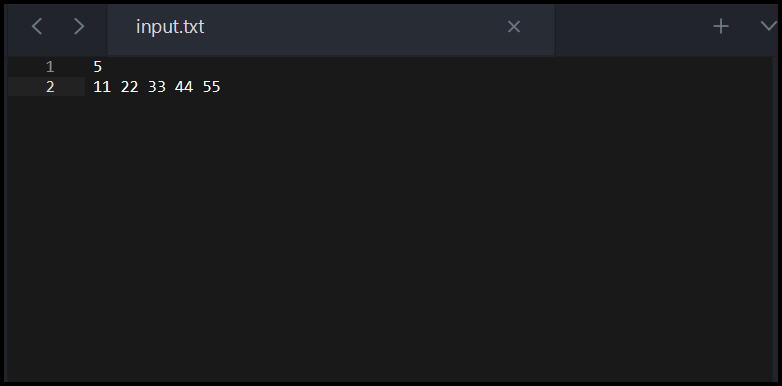output.txt fileerror.txt file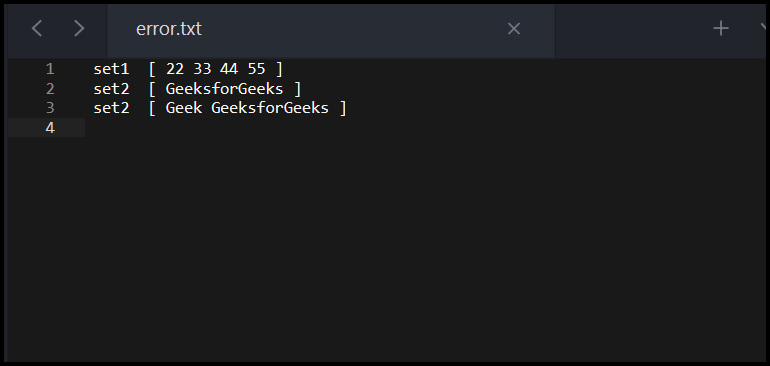Advantage of this method of debugging:

• Now there is no need to comment on each of the debug statements in the program before submitting the source code file to online judges.
• The data structure or STL elements would be printed in a separate file (error.txt) and desired output values would be printed in the output.txt file, making it more readable.
• In a nutshell, this can save a lot of time during a coding contest.

My Personal Notes arrow_drop_up